# 928 Halloween Cat

This cat has arrived just in time for Halloween. Find the factors that go with the clues in the grid to make this Halloween Cat puzzle a multiplication table:Print the puzzles or type the solution on this excel file: 12 factors 923-931

Now let me tell you a little about the number 928.

It is the sum of four consecutive prime numbers:
227 + 229 + 233 + 239 = 928
and the sum of eight other consecutive prime numbers:
101 + 103 + 107 + 109 + 113 + 127 + 131 + 137 = 928

It is the sum of two squares:
28² + 12² = 928
That means 928 is the hypotenuse of a Pythagorean triple:
640-672-928 which is 28² – 12², 2(28)(12), 28² + 12²

Here’s how 928 looks in a few other bases:
It’s 565 in BASE 13, because 5(169) + 6(13) + 5(1) = 928.
It’s 4A4 in BASE 14 (A is 10 base 10), because 4(196) + 10(14) + 4(1) = 928.
It’s TT in BASE 31 (T is 29 base 10), because 29(31) + 29(1) = 29(32) = 928.
It’s T0 in BASE 32, because 29(32) = 928.

• 928 is a composite number.
• Prime factorization: 928 = 2 × 2 × 2 × 2 × 2 × 29, which can be written 732 = 2⁵ × 29
• The exponents in the prime factorization are 5 and 1. Adding one to each and multiplying we get (5 + 1)(1 + 1) = 6 × 2 = 12. Therefore 928 has exactly 12 factors.
• Factors of 928: 1, 2, 4, 8, 16, 29, 32, 58, 116, 232, 464, 928
• Factor pairs: 928 = 1 × 928, 2 × 464, 4 × 232, 8 × 116, 16 × 58, or 29 × 32
• Taking the factor pair with the largest square number factor, we get √928 = (√16)(√58) = 4√58 ≈ 30.463092423.# 927 Candy Corn

Candy corn is a traditional Halloween candy.

Figure out what number goes in the top cell of the first column of this level three candy corn puzzle, and work your way down the first column, cell by cell, to make this puzzle a treat to complete.Print the puzzles or type the solution on this excel file: 12 factors 923-931

Fibonacci numbers begin with 1, 1, with the rest of the numbers in the sequence being the sum of the previous two.

Tribonacci numbers begin with 0, 0, 1 with the rest of the numbers in the sequence being the sum of the previous THREE.

The first 15 tribonacci numbers are 0, 0, 1, 1, 2, 4, 7, 13, 24, 44, 81, 149, 274, 504, 927. Thank you, Stetson.edu, for that fun fact.

• 927 is a composite number.
• Prime factorization: 927 = 3 × 3 × 103, which can be written 927 = 3² × 103
• The exponents in the prime factorization are 2 and 1. Adding one to each and multiplying we get (2 + 1)(1 + 1) = 3 × 2  = 6. Therefore 927 has exactly 6 factors.
• Factors of 927: 1, 3, 9, 103, 309, 927
• Factor pairs: 927 = 1 × 927, 3 × 309, or 9 × 103
• Taking the factor pair with the largest square number factor, we get √927 = (√9)(√103) = 3√103 ≈ 30.44667

# 921 Is This Bug Cute or Creepy?

Some bugs make creepy Halloween decorations. Other bugs, like ladybugs, might make a very cute costume.

Today’s puzzle looks like a bug, but there is no reason to run and hide from this one. Yes, it’s a level 5, so some parts of it may be tricky.

This is what you need to do to solve it: stay calm; don’t guess and check. Figure out where to put each number from 1 to 10 in both the top row and the first column so that the clues make the puzzle work like a multiplication table. Don’t write a number down unless you are absolutely sure it belongs where you’re putting it. Use logic, step by step, and this puzzle will be a treat.Print the puzzles or type the solution on this excel file: 10-factors-914-922

When you put on a Halloween costume, you may look completely different.

When a number is written in a different base, it may look completely different. For example,
921 looks like repdigit 333 in BASE 17 because 3(17²) + 3(17¹) + 3(17º) = 3(289 + 17 + 1) = 3(307) = 921
(307 is 111 in BASE 17)

921 looks like palindrome 1H1 in BASE 23 (H is 17 base 10). As you might suspect, 1(23²) + 17(23¹) + 1(23º) = 529 + 391 + 1 = 921

When it’s not written in a different base, 921 looks pretty familiar. You can tell quite quickly that it is divisible by 3:

• 921 is a composite number.
• Prime factorization: 921 = 3 × 307
• The exponents in the prime factorization are 1 and 1. Adding one to each and multiplying we get (1 + 1)(1 + 1) = 2 × 2 = 4. Therefore 921 has exactly 4 factors.
• Factors of 921: 1, 3, 307, 921
• Factor pairs: 921 = 1 × 921 or 3 × 307
• 921 has no square factors that allow its square root to be simplified. √921 ≈ 30.34798181# 920 Witches’ Cauldron

“Double, double toil and trouble;
Fire burn, and caldron bubble.”

What besides “eye of newt” goes in witches’ cauldrons? The list includes some horrifying ingredients that you can read here from one scene from Shakeaspeare’s play, MacBeth.

Instead of putting “Eye of newt, and toe of frog, Wool of bat, and tongue of dog” and so forth in today’s Halloween cauldron puzzle, I just put a bunch of asterisks.Print the puzzles or type the solution on this excel file: 10-factors-914-922

“Double, double toil and trouble;
Fire burn, and caldron bubble.”

Double 115 is 230.

Double 230 is 460.

Double 460 is 920, today’s post number.

920 is the hypotenuse of a Pythagorean triple:
552-736-920 which is (3-4-5) times 184.

920 is palindrome 767 in BASE 11 because 7(121) + 6(11) + 7(1) = 920

• 920 is a composite number.
• Prime factorization: 920 = 2 × 2 × 2 × 5 × 23, which can be written 920 = 2³ × 5 × 23
• The exponents in the prime factorization are 3, 1, and 1. Adding one to each and multiplying we get (3 + 1)(1 + 1)(1 + 1) = 4 × 2 × 2 = 16. Therefore 920 has exactly 16 factors.
• Factors of 920: 1, 2, 4, 5, 8, 10, 20, 23, 40, 46, 92, 115, 184, 230, 460, 920
• Factor pairs: 920 = 1 × 920, 2 × 460, 4 × 230, 5 × 184, 8 × 115, 10 × 92, 20 × 46, or 23 × 40
• Taking the factor pair with the largest square number factor, we get √920 = (√4)(√230) = 2√230 ≈ 20.331501776.# 918 Grim Reaper’s Scythe

Sometime on Halloween you are likely to see the Grim Reaper carrying a scythe. Together they look pretty scary. This puzzle isn’t that bad though. You should give it a try.Print the puzzles or type the solution on this excel file: 10-factors-914-922

Scythe, now that is a good word to try when playing hangman. ☺

Let me tell you about the number 918:

It is the sum of consecutive prime numbers: 457 + 461 = 918

It is the hypotenuse of a Pythagorean triple:
432-810-918, which is (8-15-17) times 54

918 looks interesting in a few other bases:

• 646 in BASE 12, because 9(144) + 4(12) + 6(1) = 918
• 330 in BASE 17, because 3(289) + 3(17) + 0(1) = 3(289 + 17) = 3(306) = 918
• 198 in BASE 26, which is the digits of 918 in a different order. Note that 1(26²) + 9(26) + 8(1) = 918
• RR in BASE 33, (R is 27 in base 10), because 27(33) + 27(1) = 27(33 + 1) = 27(34) = 918
• R0 in BASE 34, because 27(34) = 918

918 has consecutive numbers, 17 and 18, as two of its factors. That means 918 is a multiple of the 17th triangular number, 153.

• 918 is a composite number.
• Prime factorization: 918 = 2 × 3 × 3 × 3 × 17, which can be written 918 = 2 × 3³ × 17
• The exponents in the prime factorization are 1, 3, and 1. Adding one to each and multiplying we get (1 + 1)(3 + 1)(1 + 1) = 2 × 4 × 2 = 16. Therefore 918 has exactly 16 factors.
• Factors of 918: 1, 2, 3, 6, 9, 17, 18, 27, 34, 51, 54, 102, 153, 306, 459, 918
• Factor pairs: 918 = 1 × 918, 2 × 459, 3 × 306, 6 × 153, 9 × 102, 17 × 54, 18 × 51, or 27 × 34
• Taking the factor pair with the largest square number factor, we get √918 = (√9)(√102) = 3√102 ≈ 30.29851# 917 How to Solve for X with Candy

How many m&m’s are there in one fun size Halloween pack of m&m’s?

I don’t know. You don’t know. Nobody knows. That’s why these little fun size packs make the perfect UNKNOWN. For this activity, there may even be a negative number of m&m’s in a pack because I’m only using blue and orange m&m’s, and I’m letting each blue m&m equal negative one and each orange m&m equal positive one.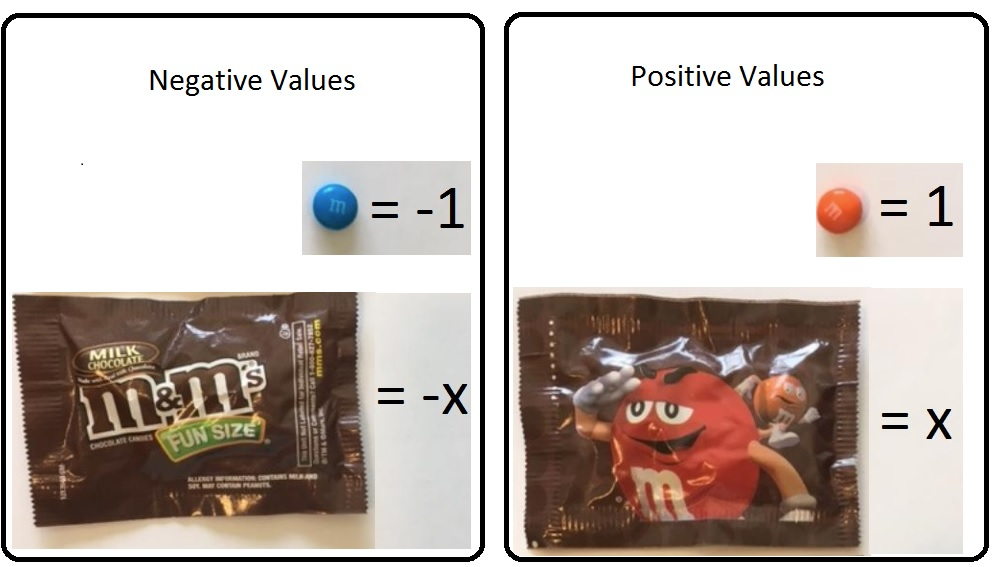I’m also letting the front side of the fun size package equal negative x, and the back side equal positive x. In algebra we often call our unknown x.

(The colors chosen don’t matter, as long as there are only two of them, and you are consistent with that color being positive or negative. The front of the package could just as easily be +1 and the back -1. Consistency is important. Choose the values you want to use and stick with them. You can also use ALL the m&m’s in a few single packs and have the side of the candy with the m be positive and the side without the m be negative. You can use the empty wrappers as x and -x.)

We can figure out how many m&m’s are in the pack by balancing an equation. The number of m&m’s in a pack is x. We will solve for x by using the very best algebra tiles in the world, m&m’s!

Besides fun size m&m’s (or Skittles or Reece’s Pieces) we need a paper balance for our equations:Click Equation Balance for a printable pdf of the paper balance.

Now let’s solve x – 3 = 5 by using the m&m’s to find x. This is how the equation balance should look to begin: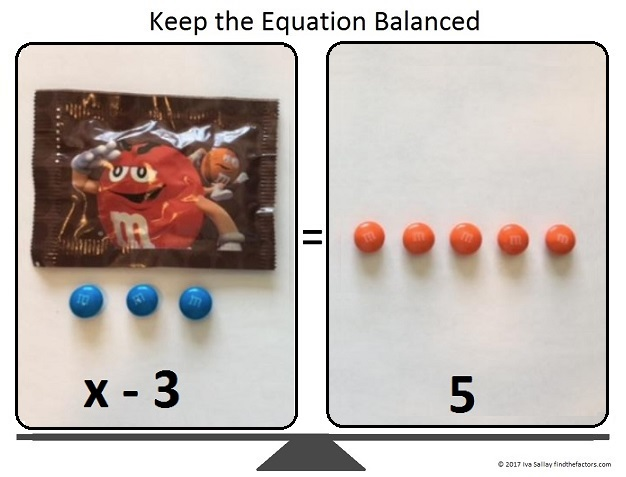We want to get the wrapper by itself, so what do we do? To keep the equation balanced, we add three (positive) orange m&m’s (one at a time) to both sides of the paper balance:Three (negative) blue m&m’s plus three (positive) orange m&m’s are equal to zero, so we can remove them.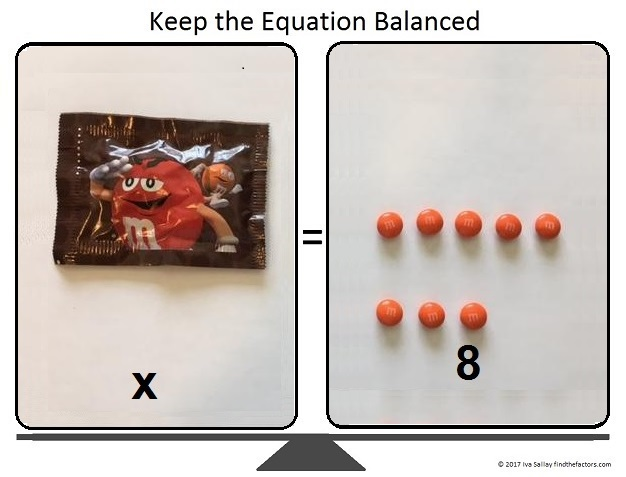Mmm. I just ate zero m&m’s, and they tasted so good! That leaves us with x = 8, so we have found x, and the equation has been solved! (If you have more equations to solve, you might want to wait to eat the m&m’s until you’re just about finished.)

Now let’s try finding x when the equation is a little more complicated, -2x = x + 12. This is how the balance should look at the beginning:We want to get all the x’s on one side so we subtract x from both sides of the equation by adding a (negative) front-facing wrapper to both sides of the balance.Since x – x = 0, we can remove the front-facing and back-facing wrappers from the right side of the equation:We can arrange the 12 candies into 3 rows of 4.Now we can divide both sides of the equation by 3.All that’s left to do is change the signs of EVERYTHING on both sides of the equation:Thus x = -4. We solved for x correctly because we kept the equation balanced every step of the way.

Now let me tell you a little bit about the number 917:

917 is the sum of five consecutive prime numbers:
173 + 179 + 181 + 191 + 193 = 917

Rearrange its digits and 917 becomes 197 in BASE 26.

• 917 is a composite number.
• Prime factorization: 917 = 7 × 131
• The exponents in the prime factorization are 1 and 1. Adding one to each and multiplying we get (1 + 1)(1 + 1) = 2 × 2 = 4. Therefore 917 has exactly 4 factors.
• Factors of 917: 1, 7, 131, 917
• Factor pairs: 917 = 1 × 917 or 7 × 131
• 917 has no square factors that allow its square root to be simplified. √917 ≈ 30.282007859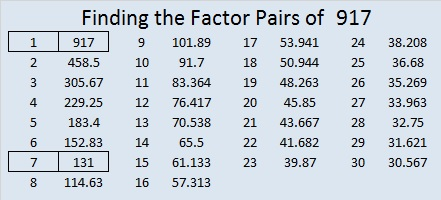# 664 The Back of a Halloween Cat’s Head

664  in base 12 is the palindrome 474. Note that 4(144) + 7(12) + 4(1)= 664.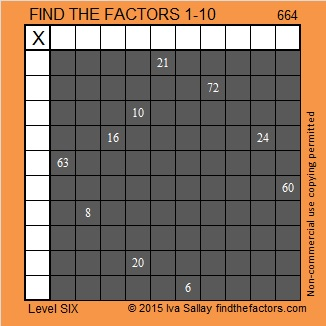Print the puzzles or type the solution on this excel file: 10 Factors 2015-10-26

—————————————————————————————————

• 664 is a composite number.
• Prime factorization: 664 = 2 x 2 x 2 x 83, which can be written 664 = (2^3) x 83
• The exponents in the prime factorization are 3 and 1. Adding one to each and multiplying we get (3 + 1)(1 + 1) = 4 x 2 = 8. Therefore 664 has exactly 8 factors.
• Factors of 664: 1, 2, 4, 8, 83, 166, 332, 664
• Factor pairs: 664 = 1 x 664, 2 x 332, 4 x 166, or 8 x 83
• Taking the factor pair with the largest square number factor, we get √664 = (√4)(√166) = 2√166 ≈ 25.768197.—————————————————————————————————Ricardo tweeted the solution: